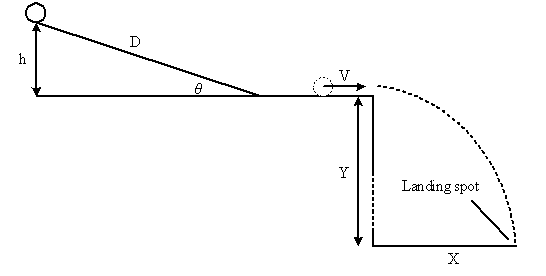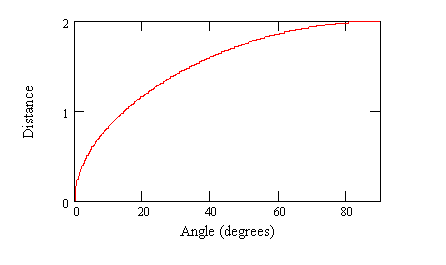# Trajectory lab

## Homework Statement

So I am doing a trajectory lab. I take a ball and release it from the ramp that is on the table and then measure the distance. The thing is when I graph the angle of the ramp vs the distance the ball has fallen I get parabola. And the correlation of parabola is 0

## Homework Equations

Parabola has correlation 0. I compared the distance I got with trajectory equation.

## The Attempt at a Solution

.[/B]
so what I found if just the angle for which the ball flies the farthest. Could I say that because the correlation is zero the lab was accurate? Or because the correlation is o i had collected bad data?
Any help appreciated.
Thanks

gneill
Mentor
Can you provide more details about the lab setup? I presume that the "distance" you measure is distance from the table to where the ball lands on the floor? Is the ball launched horizontally (say by running across part of the table) or does the ramp provide some other launch angle?

Can you provide more details about the lab setup? I presume that the "distance" you measure is distance from the table to where the ball lands on the floor? Is the ball launched horizontally (say by running across part of the table) or does the ramp provide some other launch angle?
I have a ramp o the table. The ramp is one meter from the edge of the table so I could measure the velocity of the ball. Then when it falls I measure the distance from the table. ( when it falls on the ground) I am altering the degree of the ramp, but when I graph the angle of the ramp vs the distance the ball has fallen, I get parabola. And so I am not sure about the correlation.

gneill
Mentor
This parabola that you're getting, would it happen to be "lying on its side" when you put the distance on the Y-axis and angle on the X-axis?

This parabola that you're getting, would it happen to be "lying on its side" when you put the distance on the Y-axis and angle on the X-axis?
I am getting y=-x^2 sort of shape. So when I do the correlation I get r almost zero

gneill
Mentor
I am getting y=-x^2 sort of shape. So when I do the correlation I get r almost zero

The distance the ball travels is getting shorter as the ramp angle increases?

The distance the ball travels is getting shorter as the ramp angle increases?
well, very small angle and the distance is very short. Very steep angle and the distance is also short. So the angle with the greatest distance is around 40, 50 degree.

gneill
Mentor
Does this image more or less correspond to your lab setup?gneill
Mentor
Well I must admit that I'm a bit puzzled. I would have expected the Distance vs Angle relationship to look something like this:There must be some complication involved that I'm not seeing. Perhaps after some angle the ball is sliding more than rolling down the ramp, causing it to slow down as it traverses the table due to friction with the table surface?

I'm presuming that the effects of rotational kinetic energy are meant to be ignored in this lab? (Some of the energy that the ball gains by falling through the height of the ramp will go into its linear speed, some into its rotational speed).

Is the release position (where the ball is let go) on the ramp always the same?

Well I must admit that I'm a bit puzzled. I would have expected the Distance vs Angle relationship to look something like this:

View attachment 78790

There must be some complication involved that I'm not seeing. Perhaps after some angle the ball is sliding more than rolling down the ramp, causing it to slow down as it traverses the table due to friction with the table surface?

I'm presuming that the effects of rotational kinetic energy are meant to be ignored in this lab? (Some of the energy that the ball gains by falling through the height of the ramp will go into its linear speed, some into its rotational speed).

Is the release position (where the ball is let go) on the ramp always the same?
well, from your picture when you have ramp on 90 degrees the ball goes farthest but how can it if you just throw it down and it stays on the table without actually roling down the table. Like you just take it and throw it down on the table. ( 90 degrees on the ramp)

gneill
Mentor
well, from your picture when you have ramp on 90 degrees the ball goes farthest but how can it if you just throw it down and it stays on the table without actually roling down the table. Like you just take it and throw it down on the table. ( 90 degrees on the ramp)
The picture is not meant to be taken literally at the extremes. It's based on a theoretical calculation that ignores the details of the ramp/table transition.

Of course your observation brings up a good point, did the ball begin to noticeably bounce at the interface after some angle?

The picture is not meant to be taken literally at the extremes. It's based on a theoretical calculation that ignores the details of the ramp/table transition.

Of course your observation brings up a good point, did the ball begin to noticeably bounce at the interface after some angle?
Yes,when I got to the higher degree the ball was bouncing on the table because of the transition as you mentioned as it was not very smooth, and that is where the ball lost most of the energy.

gneill
Mentor
Yes,when I got to the higher degree the ball was bouncing on the table because of the transition as you mentioned as it was not very smooth, and that is where the ball lost most of the energy.
I see. So those results are likely to be "contaminated" by factors not measured or accounted for;
Lot's of different physics coming into play.

Perhaps you can select a subset of your data where you are sure any bouncing or sliding didn't occur and the ball was traveling fast enough not to lose too large a fraction of its kinetic energy to rolling friction, and look at those results as a sub-investigation?

I'm not saying to ignore the other data! It must be presented as results of the lab, but a discussion in your error analysis or discussion of results sections might touch on this topic, and a look at the "sweet spot" results would be a good argument.

Theoretically, only taking into account simple projectile motion and energy conservation I would expect the distance to vary as the square root of the sign of the ramp angle: ## X \propto \sqrt{sin(\theta)} ##.